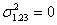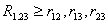# Statistics Assignment Help With Properties Of Multiple Correlation Coefficient

## 8.14.1 Properties of Multiple Correlation coefficient.

1. Multiple correlation co-efficient measures the closeness of the association between the observed values and the expected values of a variable obtained from the multiple linear regression of that variable on other variables.2. multiple correlation coefficient between observed values and expected values, when the expected values are calculated from a linear relation of the variables determined by the method of least squares, is always greater than that where expected values are calculated from any other linear combination of the variables.

3. Since R1.23 is the simple correlation between X1 and e1.23, it must lie between -1 and 1. But as seen in the above R1.23 is a non-negative quantity and we conclude that

0≤ R1.23 ≤ 1

4. if R1.23 =1 then association is perfect and all the regression residuals are zero, and as such. In the case , since X1 =e1.23 the predicted value of X1 the multiple linear regression equation of X1 on X2 and X3 may be said to be a perfect prediction formula.

5. if R1.23 =0 then all total and partial correlations involving X1  are zero. So X1 is completely uncorrelated with all the other variables in this case and the multiple regression equation fails to throw any light on the value of X 1 when X2 and X3 are known.

6. R1.23 is not less than any total correlation coefficient